Question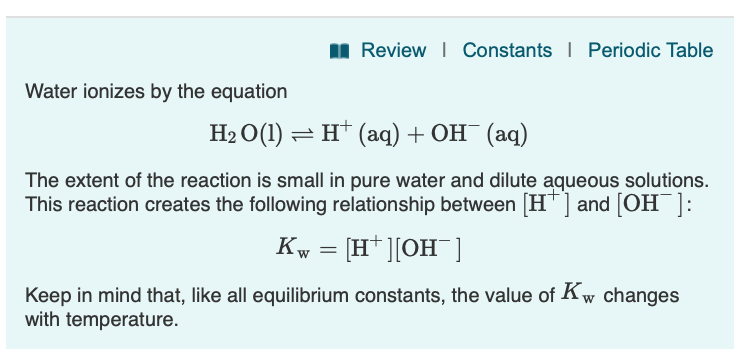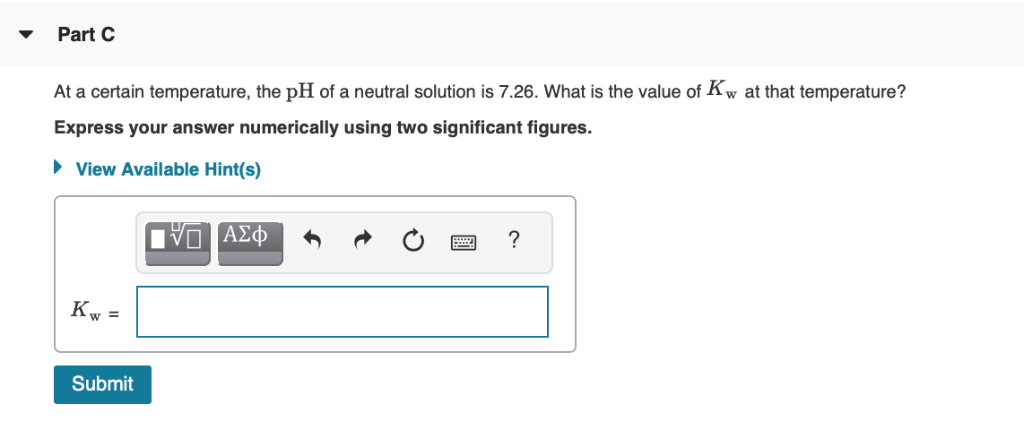Review | Constants Periodic Table Water ionizes by the equation H2 О(1) — н* (аq) + ОН (ад) The extent of the reaction is small in pure water and dilute aqueous solutions. This reaction creates the following relationship between [H ] and [OH ]: Kw 3D н'|Он ] Keep in mind that, like all equilibrium constants, the value of Kw changes with temperature
Part C At a certain temperature, the pH of a neutral solution is 7.26. What is the value of Kw at that temperature? Express your answer numerically using two significant figures View Available Hint(s) VOAD ? Kw = Submit

pH = 7.26

we have a relation between pH and [H3O+]

[H3O+] = 10-pH = 10-7.26

[H3O+] = 5.49 x 10-8 M

from Kw expression

it is clear

[H3O+] = [OH-] = 5.49 x 10-8

Kw = [H3O+][OH-]

Kw = [5.49 x 10-8][5.49 x 10-8]

Kw = 3.0 x 10-15

#### Earn Coins

Coins can be redeemed for fabulous gifts.

Similar Homework Help Questions
• ### Water ionizes by the equation H2 0()--H+ (aq) + OH-(aq) The extent of the reaction is...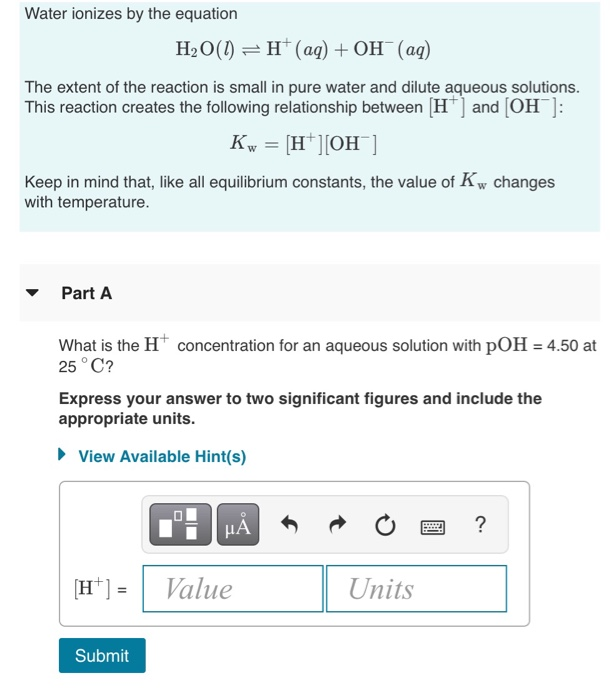Water ionizes by the equation H2 0()--H+ (aq) + OH-(aq) The extent of the reaction is small in pure water and dilute aqueous solutions. This reaction creates the following relationship between H and [OH Keep in mind that, like all equilibrium constants, the value of Kw changes with temperature. ▼ Part A What is the H concentration for an aqueous solution with pOH 4.50 at 25 ° C? Express your answer to two significant figures and include the appropriate units....

• ### Water ionizes by the equation H2O(l)⇌H+(aq)+OH−(aq) The extent of the reaction is small in pure water...

Water ionizes by the equation H2O(l)⇌H+(aq)+OH−(aq) The extent of the reaction is small in pure water and dilute aqueous solutions. This reaction creates the following relationship between [H+] and [OH−]: Kw=[H+][OH−] Keep in mind that, like all equilibrium constants, the value of Kw changes with temperature. Part A What is the H+ concentration for an aqueous solution with pOH = 3.02 at 25 ∘C?

• ### Review Constants 1 Periodic Table The concentration of OH in a sample of seawater is 1.2x10-6...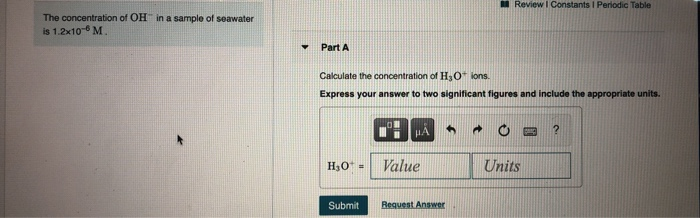Review Constants 1 Periodic Table The concentration of OH in a sample of seawater is 1.2x10-6 M Y Part A Calculate the concentration of H3O+ ions. Express your answer to two significant figures and include the appropriate units. HA ? H0+ - Value Units Submit Request Answer Review Constants ! Periodic Table Water Ionizes by the equation Part A H2O(1) H+ (aq) + OH(aq) The extent of the reaction is small in pure water and dilute aqueous solutions. This reaction...

• ### Part A the value of Ky at that temperature? Water ionizes by the equation H2O(l) =...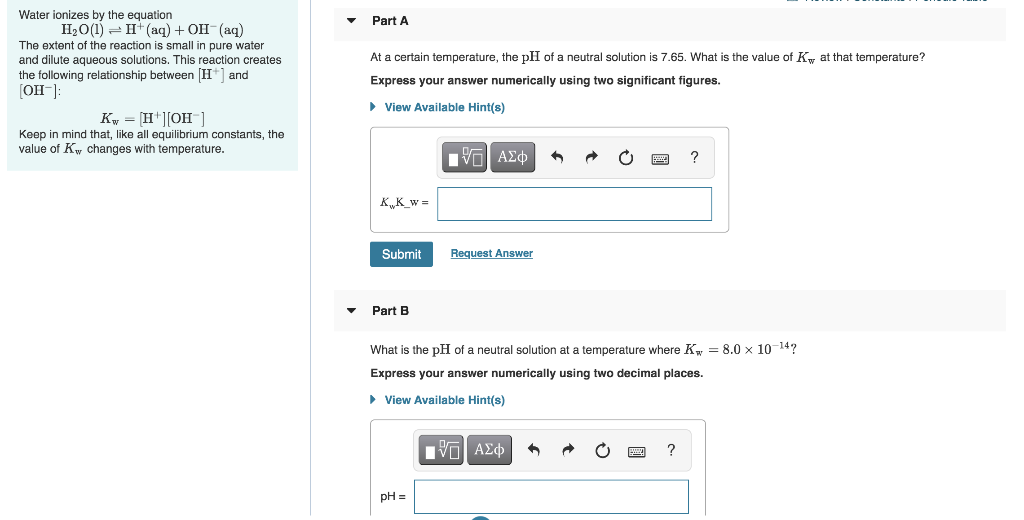Part A the value of Ky at that temperature? Water ionizes by the equation H2O(l) = 1+ (aq) + OH-(aq) The extent of the reaction is small in pure water and dilute aqueous solutions. This reaction creates the following relationship between (H+) and (OH): Ky = [H+][OH-] Keep in mind that, like all equilibrium constants, the value of Kw changes with temperature. At a certain temperature, the pH of a neutral solution is 7.65. What Express your answer numerically using...

• ### 1- Calculating [H+] for Pure Water: In a certain acidic solution at 25 ∘C, [H+] is...

1- Calculating [H+] for Pure Water: In a certain acidic solution at 25 ∘C, [H+] is 100 times greater than [OH −]. What is the value for [OH −] for the solution? In a certain acidic solution at 25 , [] is 100 times greater than [ ]. What is the value for [ ] for the solution? 1.0×10−8 M 1.0×10−7 M 1.0×10−6 M 1.0×10−2 M 1.0×10−9 M 2. ± Acid-Base Relationships in Water: Water ionizes by the equation H2O(l)⇌H+(aq)+OH−(aq)The...

• ### NA Review | Constants 1 Periodic Table pH is a logarithmic scale used to indicate the...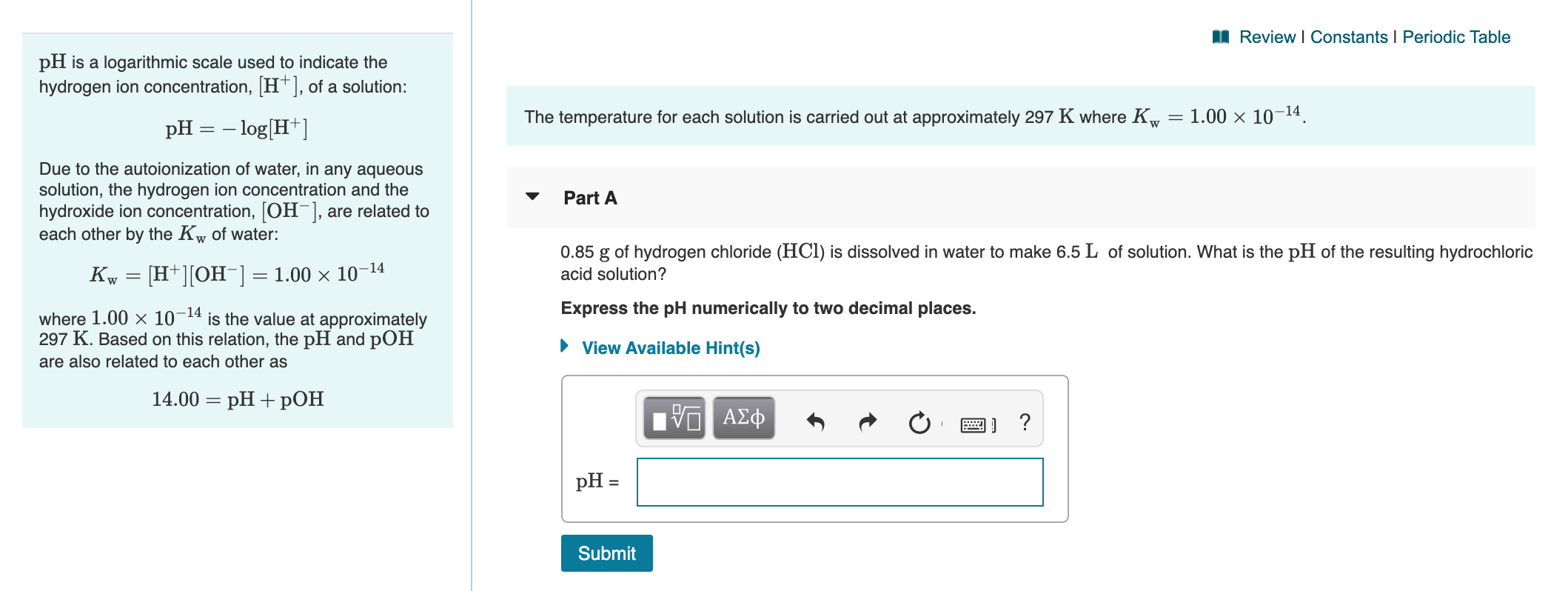NA Review | Constants 1 Periodic Table pH is a logarithmic scale used to indicate the hydrogen ion concentration, [H+], of a solution: The temperature for each solution is carried out at approximately 297 K where Kw = 1.00 x 10-14. pH = -log[H+] Due to the autoionization of water, in any aqueous solution, the hydrogen ion concentration and the hydroxide ion concentration, [OH-], are related to each other by the Kw of water: Part A Kw = [H+][OH-] =...

• ### Review I Constants I Periodic Table The equilibrium constant, K, for a redox reaction is related...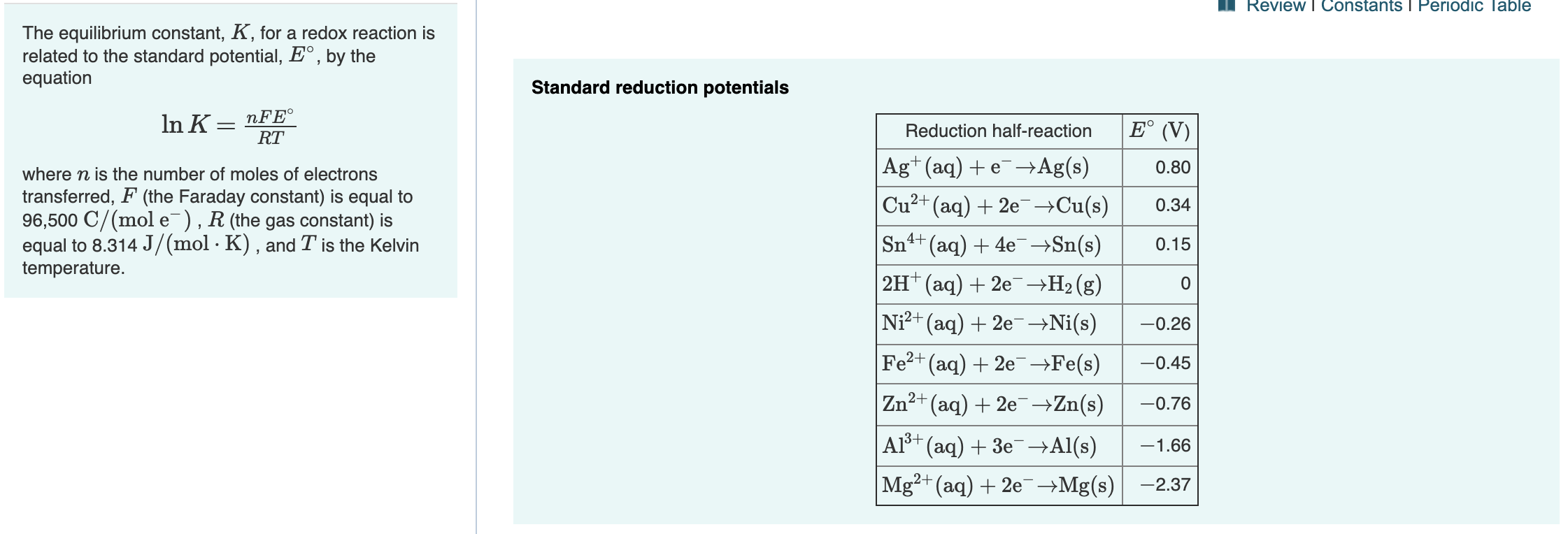Review I Constants I Periodic Table The equilibrium constant, K, for a redox reaction is related to the standard potential, E°, by the equation Standard reduction potentials nFE RT In K E° (V) Reduction half-reaction Agt(aq)eAg(s) Cu2+(aq)2eCu(s) 0.80 where n is the number of moles of electrons transferred, F (the Faraday constant) is equal to 96,500 C/(mol e), R (the gas constant) is equal to 8.314 J/(mol - K) , and T is the Kelvin temperature. 0.34 Sn4t (aq)4eSn(s) 0.15...

• ### ③ 13 of 16 Review | Constants 1 Periodic Table W Part B The following reaction...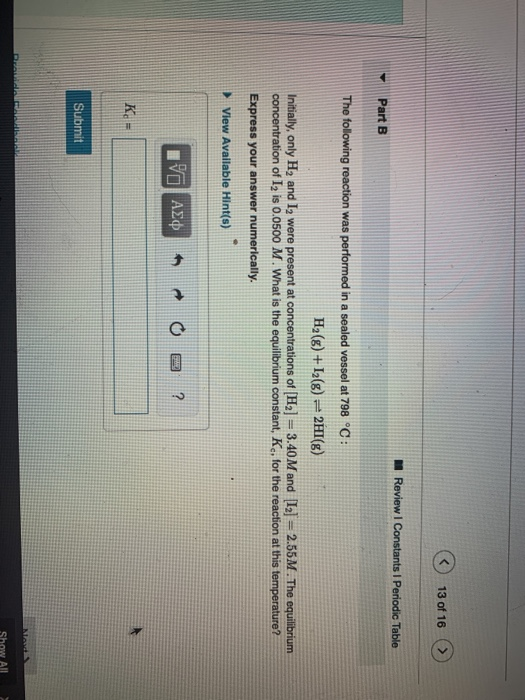③ 13 of 16 Review | Constants 1 Periodic Table W Part B The following reaction was performed in a sealed vessel at 798 °C: H2(g) +13(g) = 2HI(g) Initially, only H, and I were present at concentrations of [H2] = 3.40 M and  =2.55M. The equilibrium concentration of 12 is 0.0500 M. What is the equilibrium constant, K., for the reaction at this temperature? Express your answer numerically. View Available Hint(s) 10 AED to e ? Submit Show...

• ### MI Review | Constants Periodic Table Part A The equilibrium constant, K, of a reaction at...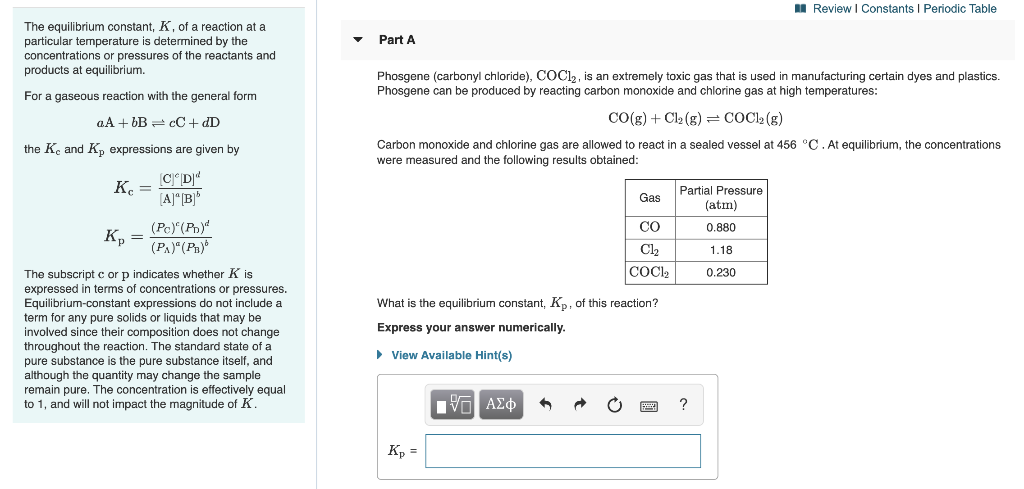MI Review | Constants Periodic Table Part A The equilibrium constant, K, of a reaction at a particular temperature is determined by the concentrations or pressures of the reactants and products at equilibrium. Phosgene (carbonyl chloride), COCl2, is an extremely toxic gas that is used in manufacturing certain dyes and plastics. Phosgene can be produced by reacting carbon monoxide and chlorine gas at high temperatures: For a gaseous reaction with the general form aA + bB =cC + dD CO(g)...